## 前言

HashMap是是Java Collections Framework的成员，位于java.util包，在JDK1.2引入。其数据存储形式是基于K-V键值对形式进行存储，HashMap中的key不能重复，允许且只能存在一个null值。如果多次put同一个key会进行值覆盖，对于value则没有限制。

``````public class TestHashMap {
public static void main(String[] args) {
HashMap<String, String> map = new HashMap<>();
map.put("hello", "world");
map.put("java", "Map");
map.put(null, null);
map.put(null, "value");

System.out.println(map);
}
}
``````

``````{null=value, java=Map, hello=world}
``````

## 源码分析

• 哈希冲突

• 数据结构特性，如数组、链表、红黑树、哈希表

• 扰动函数，如下计算索引

• 扩容机制

• put方法的流程

• 线程安全

## 什么是哈希冲突

HashMap之所以被称为Hash Map，主要是在存储元素时用到了哈希算法，又被称为散列算法。哈希算法根据设定的哈希函数和处理冲突方法将一组关键字映象到一个有限的地址区间上，由于哈希算法被计算的数据是无限的，而计算后的结果范围有限，因此总会存在不同的数据经过计算后得到的值相同，这就是哈希冲突。

HashMap中计算散列值的时候对先调用对象自身的hashCode方法，查看String类的hashCode方法：

``````public int hashCode() {
int h = hash;
if (h == 0 && value.length > 0) {
char val[] = value;
for (int i = 0; i < value.length; i++) {
h = 31 * h + val[i];
}
hash = h;
}
return h;
}
``````

``````ABCDEa123abc --> 1001110110110110101011101110
ABCDFB123abc --> 1001110110110110101011101110
``````

## 构造方法

HashMap提供了多个构造方法，其中比较重要的一个构造方法需要了解：指定了初始化容量和加载因子。

``````public HashMap(int initialCapacity, float loadFactor) {
if (initialCapacity < 0)
throw new IllegalArgumentException("Illegal initial capacity: " +
initialCapacity);
if (initialCapacity > MAXIMUM_CAPACITY)
initialCapacity = MAXIMUM_CAPACITY;
throw new IllegalArgumentException("Illegal load factor: " +
this.threshold = tableSizeFor(initialCapacity);
}
``````

HashMap传入的initialCapacity参数，并没有用来记录其容量的大小，而是用来计算阈值，因为在达到阈值时就会进行扩容操作，关于阈值的计算有如下计算方法：

``````static final int tableSizeFor(int cap) {
int n = cap - 1;
n |= n >>> 1;
n |= n >>> 2;
n |= n >>> 4;
n |= n >>> 8;
n |= n >>> 16;
return (n < 0) ? 1 : (n >= MAXIMUM_CAPACITY) ? MAXIMUM_CAPACITY : n + 1;
}
``````

``````2 --> 2
4 --> 4
10 --> 16
17 --> 32
``````

**说明：**在构造方法中`this.threshold = tableSizeFor(initialCapacity);`这一行代码，将计算的结果赋值给阈值，在使用指定初始化容量的构造方法时，首次扩容会把这个计算结果赋值给HashMap的容量。

## 数据结构

HashMap中用的数据结构是哈希表，并不是具体单一的某种结构，而是复合了单一类型的数据结构，因此想要理解其实现原理，就需要先知道一些基本概念。

### 常见数据结构

#### 数组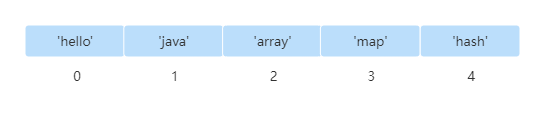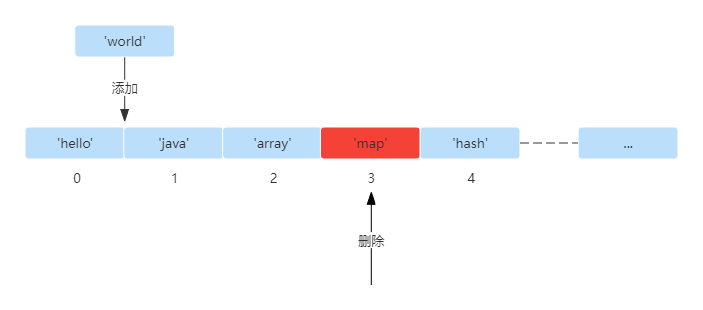#### 链表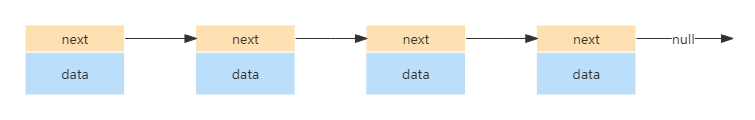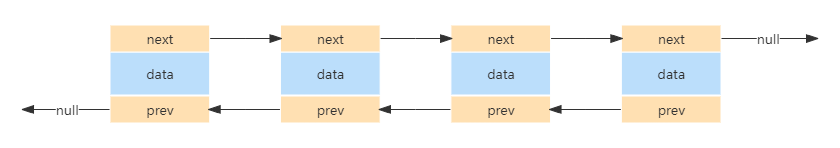#### 红黑树

1. 节点是红色或黑色。

2. 根节点是黑色。

3. 所有叶子节点都是黑色的空节点。(叶子节点是NIL节点或NULL节点)

4. 每个红色节点的两个子节点都是黑色节点。(从每个叶子节点到根的所有路径上不能有两个连续的红色节点)

5. 从任一节点到其每个叶子节点的所有路径都包含相同数目的黑色节点。

### HashMap存储结构

#### 哈希表

HashMap在JDK1.8中的底层数据结构使用的是哈希表，即`数组+链表`或者`数组+红黑树`，是一个复合结构，可以充分利用数组、链表、红黑树的特点。每个数组下标位置存储不止一个元素，会存储多个元素，因此每个下标空间可以被称为一个bucket(桶)，哈希表结构示意如图所示：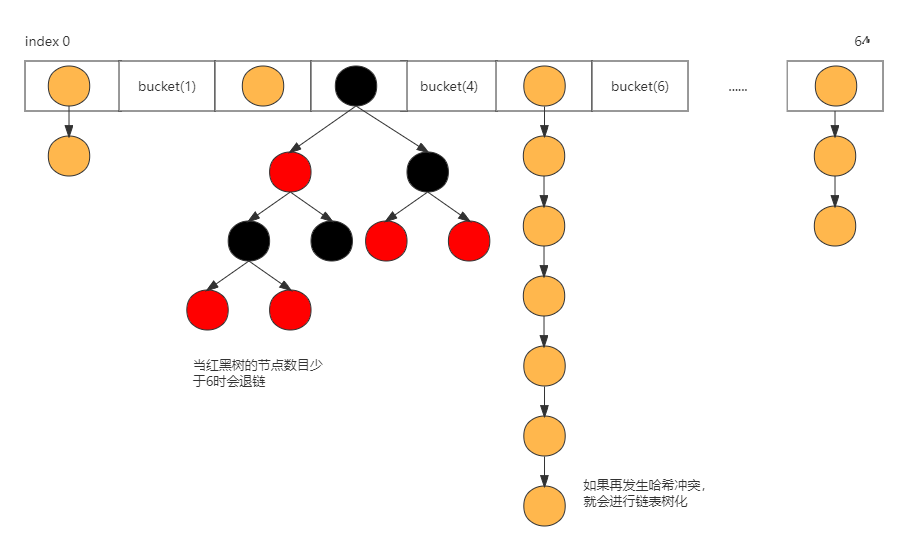#### 链表节点

``````// HashMap普通链表节点，单向链表
static class Node<K,V> implements Map.Entry<K,V> {
final int hash;
final K key;
V value;
Node<K,V> next;

Node(int hash, K key, V value, Node<K,V> next) {
this.hash = hash;
this.key = key;
this.value = value;
this.next = next;
}
}
``````

#### 红黑树节点

``````static class Entry<K,V> extends HashMap.Node<K,V> {
Entry<K,V> before, after;
Entry(int hash, K key, V value, Node<K,V> next) {
super(hash, key, value, next);
}
}

// HashMap红黑树节点，继承自普通Node节点
static final class TreeNode<K,V> extends LinkedHashMap.Entry<K,V> {
TreeNode<K,V> parent;  // red-black tree links
TreeNode<K,V> left;
TreeNode<K,V> right;
TreeNode<K,V> prev;    // needed to unlink next upon deletion
boolean red;
TreeNode(int hash, K key, V val, Node<K,V> next) {
super(hash, key, val, next);
}
}
``````

• 数组：哈希表中每一个数组的位置不止存储一个元素，可以看作一个bucket(桶)，在数组位置进行元素查找时间复杂度为O(1)。
• 链表：查找的时间复杂度为O(n)，这种情况下HashMap的综合时间复杂度为O(1)+O(n)=O(n)。
• 红黑树：查找的时间复杂度为O(logN)，该情况下HashMap的时间复杂度为O(1)+O(logN)=O(logN)。

HashMap中会采用数组+链表还是数组+红黑树的结构，取决于存储元素的多少和一些基本初始参数定义，在源码中初始化了如下重要的成员变量：

``````// HashMap中存储散列链表的数组，长度必须是2的幂
transient Node<K,V>[] table;

//默认初始容量 - 必须是 2 的幂。
static final int DEFAULT_INITIAL_CAPACITY = 1 << 4; // aka 16

//最大容量，如果一个更高的值由任何一个带参数的构造函数隐式指定时使用。必须是 2 <= 1<<30 的幂。
static final int MAXIMUM_CAPACITY = 1 << 30;

//构造函数中未指定时使用的负载因子。
static final float DEFAULT_LOAD_FACTOR = 0.75f;

//链表树化阙值： 默认值为 8 。
//表示在一个node(Table)节点下的值的个数大于8时候，会将链表转换成为红黑树。
static final int TREEIFY_THRESHOLD = 8;

//红黑树链化阙值： 默认值为 6 。
//表示在进行扩容期间，单个Node节点下的红黑树节点的个数小于6时候，会将红黑树转化成为链表。
static final int UNTREEIFY_THRESHOLD = 6;

//最小树化阈值，tab.length超过才会进行树化(为了防止前期阶段频繁扩容和树化过程冲突)。
static final int MIN_TREEIFY_CAPACITY = 64;
``````

## 泊松分布

Poisson分布，是一种统计与概率学里常见到的离散概率分布，由法国数学家西莫恩·德尼·泊松(Siméon-Denis Poisson)在1838年时发表。关于泊松分布，在源码中有如下解释：

Because TreeNodes are about twice the size of regular nodes, we use them only when bins contain enough nodes to warrant use (see TREEIFY_THRESHOLD). And when they become too small (due to removal or resizing) they are converted back to plain bins. In usages with well-distributed user hashCodes, tree bins are rarely used. Ideally, under random hashCodes, the frequency of nodes in bins follows a Poisson distribution (http://en.wikipedia.org/wiki/Poisson_distribution) with a parameter of about 0.5 on average for the default resizing threshold of 0.75, although with a large variance because of resizing granularity. Ignoring variance, the expected occurrences of list size k are
(exp(-0.5) * pow(0.5, k) / factorial(k)).
The first values are:
0: 0.60653066
1: 0.30326533
2: 0.07581633
3: 0.01263606
4: 0.00157952
5: 0.00015795
6: 0.00001316
7: 0.00000094
8: 0.00000006
more: less than 1 in ten million

## 如何计算数组下标

HashMap中如何在put时确定一个key的存储位置，并且可以在get时快速找到这个key？这决定了HashMap的性能。HashMap通过key的hashcode与Map的数组长度进行位运算来确定某个key存储bucket索引的位置。
HashMap在JDK1.7中关于下标如何计算有如下代码：

``````/**
* Returns index for hash code h.
*/
static int indexFor(int h, int length) {
// assert Integer.bitCount(length) == 1 : "length must be a non-zero power of 2";
return h & (length-1);
}
``````

``````// n为tab的length
tab[i = (n - 1) & hash]
``````

``````15 --> 0000 1111
31 --> 0001 1111
63 --> 0011 1111
``````

``````public static void testHashIndex() {
// 0000 1111    --> 15
// hello --> 101111010010001100011010010 & 1111 = 0010 --> 2
// redis --> 110011101011110010101111011 & 1111 = 1011 --> 11
// hashmap --> 101001100100111010000110001110 & 1111 = 1110 -> 14
List<String> strings = Arrays.asList("hello", "redis", "hashmap");
for (String string : strings) {
System.out.println(string.hashCode() + " --> " +
Integer.toBinaryString(string.hashCode()) + " & " +
Integer.toBinaryString(15) + " = " +
(string.hashCode() & 15));
}
}
``````

``````99162322 --> 101111010010001100011010010 & 1111 = 2
108389755 --> 110011101011110010101111011 & 1111 = 11
697541006 --> 101001100100111010000110001110 & 1111 = 14
``````

## 扰动函数

``````static final int hash(Object key) {
int h;
return (key == null) ? 0 : (h = key.hashCode()) ^ (h >>> 16);
}
``````

Computes key.hashCode() and spreads (XORs) higher bits of hash to lower. Because the table uses power-of-two masking, sets of hashes that vary only in bits above the current mask will always collide. (Among known examples are sets of Float keys holding consecutive whole numbers in small tables.) So we apply a transform that spreads the impact of higher bits downward. There is a tradeoff between speed, utility, and quality of bit-spreading. Because many common sets of hashes are already reasonably distributed (so don’t benefit from spreading), and because we use trees to handle large sets of collisions in bins, we just XOR some shifted bits in the cheapest possible way to reduce systematic lossage, as well as to incorporate impact of the highest bits that would otherwise never be used in index calculations because of table bounds.

HashMap中的关于索引计算是调用最为频繁的方法了，如果频繁出现哈希冲突，那么对于其性能也会有很大的影响，而通常用于作为key的String类型本身就有机率发生哈希冲突，再结合HashMap中索引计算的方法使得高位无法参与计算，(h = key.hashCode()) ^ (h >>> 16)这行代码是为了对hashCode进行扰动计算，防止不同hashCode的高位不同但低位相同导致的哈希冲突。

``````public static void compareDisturbanceIndex() {
String key = "星光不问赶路人，时光不负有心人";
int threshold = 16;
System.out.println("[NoDisturbance] \r\n hashcode --> " + Integer.toBinaryString(key.hashCode())
+ "\r\n index    --> " + (15 & key.hashCode()));

System.out.println("[Disturbance]   \r\n hashcode --> " + Integer.toBinaryString(key.hashCode())
+ "\r\n >>>      --> " + Integer.toBinaryString(key.hashCode() >>> 16)
+ "\r\n ^        --> " + Integer.toBinaryString((key.hashCode() ^ (key.hashCode() >>> 16)))
+ "\r\n index    --> " + (15 & (key.hashCode() ^ (key.hashCode() >>> 16))));
}
``````

``````[NoDisturbance]
hashcode --> 111110110101000001001000100101
index    --> 5

[Disturbance]
hashcode --> 111110110101000001001000100101
>>> 16   --> 11111011010100
^        --> 111110110101000010110011110001
index    --> 1
``````

``````package com.starsray.test.hashmap;

import java.util.HashMap;
import java.util.Map;
import java.util.Random;

public class TestHashMapDisturbance {

public static int disturbanceIndex(String key, int size) {
return (size - 1) & (key.hashCode() ^ (key.hashCode() >>> 16));
}

public static int index(String key, int size) {
return (size - 1) & key.hashCode();
}

public static void testIndex(int times) {
int threshold = 256;

Map<Integer, Integer> map = new HashMap<>();
Map<Integer, Integer> disMap = new HashMap<>();

for (int i = 0; i < times; i++) {
String randomKey = getRandomKey();

int index = index(randomKey, threshold);
if (map.containsKey(index)) {
Integer count = map.get(index);
map.put(index, ++count);
} else {
map.put(index, 1);
}

int disIndex = disturbanceIndex(randomKey, threshold);
if (disMap.containsKey(disIndex)) {
Integer count = disMap.get(disIndex);
disMap.put(disIndex, ++count);
} else {
disMap.put(disIndex, 1);
}
}
System.out.println("map index:");
map.forEach((k, v) -> System.out.println(k + "," + v));
System.out.println("map disturbance index:");
disMap.forEach((k, v) -> System.out.println(k + "," + v));
}

public static String getRandomKey() {
int len = (int) (Math.random() * 20 + 5);
Random random = new Random();
StringBuilder sb = new StringBuilder();
for (int i = 0; i < len; i++) {
sb.append(str.charAt(random.nextInt(str.length())));
}
return sb.toString();
}

public static void main(String[] args) {
testIndex(100_000);
}
}
``````

## put方法的流程

HashMap的put方法是实现最为复杂的，查看HashMap的put方法源码，其内部调用了一个私有的putVal方法：

``````public V put(K key, V value) {
return putVal(hash(key), key, value, false, true);
}
``````

• hash(key)：经过扰动函数计算后的key的hash值，尽可能让key自身hashcode高位参与元算，提高散列效果
• key：存放的key值
• value：存放的value值
• onlyIfAbsent：是否覆盖现有的值，默认为false，即相同key后put的值会替换先put的值

``````final V putVal(int hash, K key, V value, boolean onlyIfAbsent,
boolean evict) {
Node<K,V>[] tab; Node<K,V> p; int n, i;
// 首次添加元素，哈希表的容量即HashMap未进行初始化，容量为null
if ((tab = table) == null || (n = tab.length) == 0)
// 对哈希表进行扩容，并且将哈希表扩容后的长度赋值给n，此处的n为2的幂次数
n = (tab = resize()).length;
// 根据当前key扰动后的哈希值进行下标位置计算，如果对应下标处的节点为空
// 当前put的元素会创建为一个K-V结构，并存储在tab[i]位置处
if ((p = tab[i = (n - 1) & hash]) == null)
tab[i] = newNode(hash, key, value, null);
// 这里说明已经发生了哈希碰撞，哈希表中存储的元素要开始进行链化或者树化
else {
Node<K,V> e; K k;
// 这里的p指向当前key在哈希表中i索引处的节点，也就是发生碰撞的节点
// 如果p的哈希值和传入的哈希值相等或则p.key等于传入的key，或者key不为null的场景下
// 内容也一直，说明冲突的是同一个key,当前索引的节点p用e临时保存。
if (p.hash == hash &&
((k = p.key) == key || (key != null && key.equals(k))))
e = p;
// 如果当前的节点p是红黑树节点，说明整个哈希表已经树化，后面的节点也将使用红黑树处理
else if (p instanceof TreeNode)
// this为当前HashMap对象实例，tab为当前HashMap对象中的哈希表
e = ((TreeNode<K,V>)p).putTreeVal(this, tab, hash, key, value);
else {
// 使用临时节点e，从当前节点p开始进行链表的遍历
// 直到循环链表的尾节点，停止循环
for (int binCount = 0; ; ++binCount) {
// 如果当前节点的next指向为null，直接新建节点使用尾插法追加
if ((e = p.next) == null) {
p.next = newNode(hash, key, value, null);
// 从0到7，如果循环次数达到阈值，会进一步判断进行扩容还是使用红黑树
if (binCount >= TREEIFY_THRESHOLD - 1) // -1 for 1st
// 在treeifyBin方法中当table的长度小于64时会进行扩容
// 当table的长度超过64，并且每个下标处的元素超过8才会进行树化操作
treeifyBin(tab, hash);
break;
}
// 遍历过程中如果链表某个节点的key和当前操作key一致时，停止遍历
// 取出当前节点，并且此时临时节点e指向传入key所对应的节点，用于
// 下一步返回节点的旧值和新值的赋值
if (e.hash == hash &&
((k = e.key) == key || (key != null && key.equals(k))))
break;
// 每次遍历后p指向新节点，驱动链表移动
p = e;
}
}
if (e != null) { // existing mapping for key
V oldValue = e.value;
// 如果覆盖或者当前oldValue为null，使用新值覆盖旧值
if (!onlyIfAbsent || oldValue == null)
e.value = value;
afterNodeAccess(e);
// 返回节点的旧值
return oldValue;
}
}
// 记录HashMap被修改的次数
++modCount;
// 如果当前size大于阈值，进行扩容
if (++size > threshold)
resize();
afterNodeInsertion(evict);
// 首次put元素时返回的旧值为null
return null;
}
``````
• HashMap在初始化时并不会初始化容量，在调用`put`方法时才会去初始化容量，在`put`时先使用扰动函数对`key``hashcode`进行二次计算，再根据数组的`length - 1`进行运算确定元素的下标位置，根据当前下标处是否有元素，可分为发生哈希冲突和未冲突场景：
• 未发生哈希冲突：
• 首次：先调用`resize()`方法进行扩容，初始化table的容量，确定`bucket`的位置，存放元素。
• 非首次：这种场景同首次添加类似，只不过少了初始化容量的步骤，直接计算元素`bucket`的位置进行存放。
• 发生哈希冲突：发现新添加的元素指向的bucket位置不为空，使用临时节点e指向该位置的首个Node
• 红黑树：如果当前Node是红黑树节点(TreeNode)使用红黑树的`putTreeVal`方法进行元素添加。
• 链表：对链表进行遍历，使用尾插法进行元素添加，如果新添加的key与链表中的key一致，则取出旧值返回，使用新值替换掉旧值。在链表遍历时会判断链表元素长度，如果大于8个元素，会在`treeifyBin`方法中进行判断，如果table长度小于64会进行扩容，超过链表会进行红黑树转变。

• 首次进行元素添加，即table == null || table.length ==0，调用resize方法初始化容量。
• 发生哈希冲突，当链表满足TREEIFY_THRESHOLD = 8 && table.length < MIN_TREEIFY_CAPACITY会触发resize进行扩容。
• 当put元素后的容量，size > threshold会触发扩容

## 扩容机制

HashMap在存储元素数量达到阈值时会对数组的长度进行扩容，扩容的实现主要在`resize()`方法中，源码实现中可以将代码看作两部分，查看源码：

``````final Node<K,V>[] resize() {
// 第一部分：计算哈希表table的阈值和新的要扩容的容量
Node<K,V>[] oldTab = table;
int oldCap = (oldTab == null) ? 0 : oldTab.length;
int oldThr = threshold;
int newCap, newThr = 0;
......省略代码

// 第二部分：根据第一部分计算的新容量newCap扩容，并对元素进行迁移
@SuppressWarnings({"rawtypes","unchecked"})
Node<K,V>[] newTab = (Node<K,V>[])new Node[newCap];
table = newTab;
......省略代码
return newTab;
}
``````

``````import java.util.Arrays;
import java.util.HashMap;
import java.util.HashSet;
import java.util.Random;

public class TestResize {

public static void main(String[] args) {
int cap = 2000_00;
int cycle = (int) (cap * 0.75) - 1;
String[] arr = createArr(cycle);
System.out.println(arr.length);
HashSet<String> set = new HashSet<>(Arrays.asList(arr));
System.out.println(set.size());

HashMap<String, Object> initMap = new HashMap<>(cap);
HashMap<String, Object> map = new HashMap<>();

work(cycle, arr, initMap);
work(cycle, arr, map);
}

private static void work(int cycle, String[] arr, HashMap<String, Object> map) {
long start = System.currentTimeMillis();
for (int i = 0; i < cycle; i++) {
map.put(arr[i], getRandomKey());
}
long end = System.currentTimeMillis();
System.out.println(end - start);
}

static String[] createArr(int cycle) {
String[] arr = new String[cycle];
for (int i = 0; i < cycle; i++) {
arr[i] = getRandomKey();
}
return arr;
}

public static String getRandomKey() {
int len = (int) (Math.random() * 25 + 5);
Random random = new Random();
StringBuilder sb = new StringBuilder();
for (int i = 0; i < len; i++) {
sb.append(str.charAt(random.nextInt(str.length())));
}
return sb.toString();
}
}
``````

### 计算容量和阈值

HashMap在元素达到阈值时会进行扩容，其中前半段部分代码主要是计算如何扩容，如下所示：

``````final Node<K,V>[] resize() {
// 声明临时变量oldTable指向原来的数组，oldThr为原来的阈值，oldCap为原来数组长度或0
Node<K,V>[] oldTab = table;
int oldCap = (oldTab == null) ? 0 : oldTab.length;
int oldThr = threshold;
// 声明新的容量和阈值进行扩容
int newCap, newThr = 0;

if (oldCap > 0)
// 如果oldCap>=MAXIMUM_CAPACITY=2^30，直接定义阈值threshold为2^31-1，并且返回
if (oldCap >= MAXIMUM_CAPACITY) {
threshold = Integer.MAX_VALUE;
return oldTab;
}
// 当新的容量newCap小于MAXIMUM_CAPACITY，并且
// 旧的容量oldCap大于默认容量16时，进行扩容时阈值newThr也加倍
else if ((newCap = oldCap << 1) < MAXIMUM_CAPACITY &&
oldCap >= DEFAULT_INITIAL_CAPACITY)
newThr = oldThr << 1; // double threshold
}
else if (oldThr > 0) // initial capacity was placed in threshold
// 需要注意这里的注释，JDK中一般添加注释的地方都需要特别关注
// 前面提到构造方法时，在指定构造方法时，把计算table的size赋值给了threshold，
// 在首次扩容并进行put时，会把tableSizeFor方法的计算结果通过oldThr赋值给newCap
newCap = oldThr;
else {   // zero initial threshold signifies using defaults
// 首次进行put，使用默认的参数给newCap=16，newThr=16*0.75=12进行赋值
newCap = DEFAULT_INITIAL_CAPACITY;
}
// 在指定初始化容量时，进行扩容时会进行阈值的计算
if (newThr == 0) {
// 计算阈值
float ft = (float)newCap * loadFactor;
// 判断是否达到最大值进行阈值取值
newThr = (newCap < MAXIMUM_CAPACITY && ft < (float)MAXIMUM_CAPACITY ?
(int)ft : Integer.MAX_VALUE);
}
// 经过计算，最终确定HashMap中threshold的值
threshold = newThr;
...
}
``````

HashMap在进行resize时会根据不同场景进行分别判断，根据是否已经进行初始化，未进行初始化时采用默认参数进行阈值和容量的计算，在指定初始化参数时或者已经有元素时会判断扩容后的容量是否达到最大阈值。

### 元素迁移

`resize`方法的前半部分，确定了新的阈值`newThr`和容量`newCap`。接下来需要对元素校验是否需要进行迁移。

``````final Node<K,V>[] resize() {
...省略部分

// 利用前面已经计算好的容量newCap创建一个新的数组newTable
Node<K,V>[] newTab = (Node<K,V>[])new Node[newCap];
// 将成员变量table指向新的数组引用，进行替换
table = newTab;
// 如果旧的数组不为null，说明已经进行过扩容，非首次，可能进行元素迁移，如果是首次进入
// 直接返回初始化的newTab
if (oldTab != null) {
// 外层对数组进行循环遍历，遍历的边界为扩容前的数组容量，覆盖到数组内的所有元素
for (int j = 0; j < oldCap; ++j) {
// 声明临时变量e
Node<K,V> e;
// 对原数组的每个下标处的元素进行遍历，如果数组对应下标处元素不为空进一步处理
if ((e = oldTab[j]) != null) {
// 把数组对应下标为j且不为空处的元素置为null
oldTab[j] = null;
// 如果e.next == null，说明e所处的下标j位置只有单个元素
if (e.next == null)
// 在新数据中对e重新进行下标判定
newTab[e.hash & (newCap - 1)] = e;
else if (e instanceof TreeNode)
// 如果当前节点是红黑树节点进行特殊操作
((TreeNode<K,V>)e).split(this, newTab, j, oldCap);
else { // preserve order
// 其他情况说明该位置处为链表，需要对链表进行操作

// 声明了五个临时变量
Node<K,V> loHead = null, loTail = null;
Node<K,V> hiHead = null, hiTail = null;
Node<K,V> next;
// 生成链表
do {
next = e.next;
// 这里使用e.hash与扩容前的容量进行与运算，如果与运算的最终结果为0
// 说明扩容后的元素不需要进行迁移，否则元素需要进行迁移。
// 这里是一个巧妙的设计，i=h & (len - 1)，即i= h & 2^n - 1
// 扩容后是否迁移由2^n-1的高位决定，而2^n-1的高位与2^n的高位一致
// 因此通过h & oldCap可以判断元素在扩容后是否需要进行迁移
// 0 1 0000 --> 16 0 0 1111 --> 15
// 1 0 0000 --> 32 0 1 1111 --> 31

// 无论元素是否需要迁移，接下来都需要重新构建链表

// 元素bucket位置不迁移，组成新的链表
if ((e.hash & oldCap) == 0) {
// 如果链表的尾为null，说明头节点为null，临时节点e
if (loTail == null)
else
// 尾节点不为空，当前临时节点e为原来尾节点的下一个节点
loTail.next = e;
// 第一次遍历首、尾节点都指向e，非首次遍历loTail指向新的尾节点
// 驱动链表追加元素
loTail = e;
}
// 元素bucket位置需要迁移，组成新的链表
else {
// 同上，高位需要迁移位置的链表遍历追加，尾插法
if (hiTail == null)
else
hiTail.next = e;
hiTail = e;
}
} while ((e = next) != null); // 直到遍历到链表的尾部
// 判断链表存放的位置，将头节点存放在bucket位置
if (loTail != null) {
loTail.next = null;
// 低位元素不迁移，完成重组链表的整体组建
}
if (hiTail != null) {
// 高位元素迁移至索引+旧容量位置，重组链表整体迁移至新的位置
hiTail.next = null;
}
}
}
}
}
return newTab;
}
``````

JDK8中进行扩容时，没有rehash操作，数组扩容的大小为原来的2倍，恒为2的幂，扩容后元素是否迁移根据高低位进行判断。对于链表结构的bucket位置是否进行元素迁移，根据链表中元素key的hashcode与旧的容量进行位运算，由于容量为2^n，因此计算后的元素位置要么不迁移，要么迁移为当前下标+旧容量位置处，如：15–>31。

``````public static void main(String[] args) {
System.out.printf("%032d%n",Integer.parseInt(Integer.toBinaryString(15)));
System.out.printf("%032d%n",Integer.parseInt(Integer.toBinaryString(31)));
System.out.printf("%032d%n",Integer.parseInt(Integer.toBinaryString(63)));
}
``````

``````00000000000000000000000000001111
00000000000000000000000000011111
00000000000000000000000000111111
``````

``````00000000000000000000000000001111 --> 15
00000000000000000000000000011111 --> 31

00000000000000000000000000110100 --> 52

00000000000000000000000000000100 --> 15 & 52 --> i = 4
00000000000000000000000000010100 --> 31 & 52 --> i = 20
``````

## JDK7和JDK8实现的区别

JDK8相比于JDK7，HashMap主要做了如下优化：

• 数据结构：由数组+链表改成了数组+链表/红黑树，查找的时间复杂度为O(1) ~ O(logN) ~ O(n)。
• 插入方式：1.7采用头插法，头插法会使链表发生反转，多线程环境下会产生环，并且会产生死循环、数据丢失、数据覆盖的问题，1.8采用尾插法会产生数据覆盖问题。
• 扩容方式：1.7 需要对原数组中的元素进行重新 hash 定位在新数组的位置，1.8 采用更简单的判断逻辑，位置不变或索引+旧容量大小。
• 扩容时机：1.7 先判断是否需要扩容，再插入，JDK1.8 先进行插入，插入完成再判断是否需要扩容。

### 头插法和尾插法的区别

JDK7进行添加元素时调用put方法，查看源码：

``````public V put(K key, V value) {
// 如果table未进行初始化，先进行初始化
if (table == EMPTY_TABLE) {
inflateTable(threshold);
}
// 如果key为null，添加一个null-value的entry
if (key == null)
return putForNullKey(value);
// 扰动函数计算key的hashcode
int hash = hash(key);
// 计算key在table中的索引位置
int i = indexFor(hash, table.length);
// 对链表进行遍历，使用临时节点e记录table[i]，使用e=e.next进行链表驱动，从头部开始遍历
for (Entry<K,V> e = table[i]; e != null; e = e.next) {
Object k;
// 如果链表中的key与put的key相等，用新的value替换oldValue，并返回oldValue
if (e.hash == hash && ((k = e.key) == key || key.equals(k))) {
V oldValue = e.value;
e.value = value;
e.recordAccess(this);
return oldValue;
}
}

modCount++;
// 如果链表中不包含当前key，添加当前key-value组成的entry
return null;
}
``````

### 扩容实现的差别

JDK7中的扩容是在添加元素前进行扩容，扩容大小为原数组长度的2倍。

``````void addEntry(int hash, K key, V value, int bucketIndex) {
// 当size>阈值，并且table索引处的元素不为空会触发扩容
if ((size >= threshold) && (null != table[bucketIndex])) {
// 扩容的大小为原来table的2倍
resize(2 * table.length);
// 扩容后对key的hashcode重新计算
hash = (null != key) ? hash(key) : 0;
// 重新确定key在table中的索引位置
bucketIndex = indexFor(hash, table.length);
}
// 创建新的节点
createEntry(hash, key, value, bucketIndex);
}

// 扩容实现
void resize(int newCapacity) {
// 声明临时变量oldTable指向当前table
Entry[] oldTable = table;
// 声明临时变量oldCapacity记录当前table的长度
int oldCapacity = oldTable.length;
// 如果当前的容量达到了最大值MAXIMUM_CAPACITY，阈值为Integer.MAX_VALUE，不再扩容
if (oldCapacity == MAXIMUM_CAPACITY) {
threshold = Integer.MAX_VALUE;
return;
}

// 创建一个指定长度为newCapacity的数组
Entry[] newTable = new Entry[newCapacity];
// 进行元素迁移，执行initHashSeedAsNeeded方法判断是否需要rehash
transfer(newTable, initHashSeedAsNeeded(newCapacity));
// 将成员变量table指向扩容后的newTable
table = newTable;
// 计算新的阈值
threshold = (int)Math.min(newCapacity * loadFactor, MAXIMUM_CAPACITY + 1);
}

// 添加元素节点
void createEntry(int hash, K key, V value, int bucketIndex) {
Entry<K,V> e = table[bucketIndex];
// 采用头插法，使得当前的链表头节点变成新插入节点的后继节点
table[bucketIndex] = new Entry<>(hash, key, value, e);
size++;
}
``````

``````void transfer(Entry[] newTable, boolean rehash) {
// 声明newCapacity记录新的容量值
int newCapacity = newTable.length;
// 外层对table中Entry进行遍历，即数组遍历
for (Entry<K,V> e : table) {
// 内层对table对应位置的链表进行遍历，直到遍历到链表的尾部
while(null != e) {
// 声明next记录e的下一个节点用于接下来的遍历
Entry<K,V> next = e.next;
// 判断是否需要对key重新进行扰动函数hashcode计算
if (rehash) {
e.hash = null == e.key ? 0 : hash(e.key);
}
// 根据hashcode计算下标位置
int i = indexFor(e.hash, newCapacity);
// 对链表进行操作，e的next指向扩容后newTable[i]的位置
// 如果i位置没有元素，e.next=null
e.next = newTable[i];
// newTable在i处的节点指向e，元素e被头插入新的下标位置，完成迁移
newTable[i] = e;
// 继续驱动链表遍历
e = next;
}
}
}
``````

``````final int hash(Object k) {
int h = hashSeed;
if (0 != h && k instanceof String) {
return sun.misc.Hashing.stringHash32((String) k);
}

h ^= k.hashCode();

// This function ensures that hashCodes that differ only by
// constant multiples at each bit position have a bounded
// number of collisions (approximately 8 at default load factor).
h ^= (h >>> 20) ^ (h >>> 12);
return h ^ (h >>> 7) ^ (h >>> 4);
}
``````

### 多线程扩容为什么不安全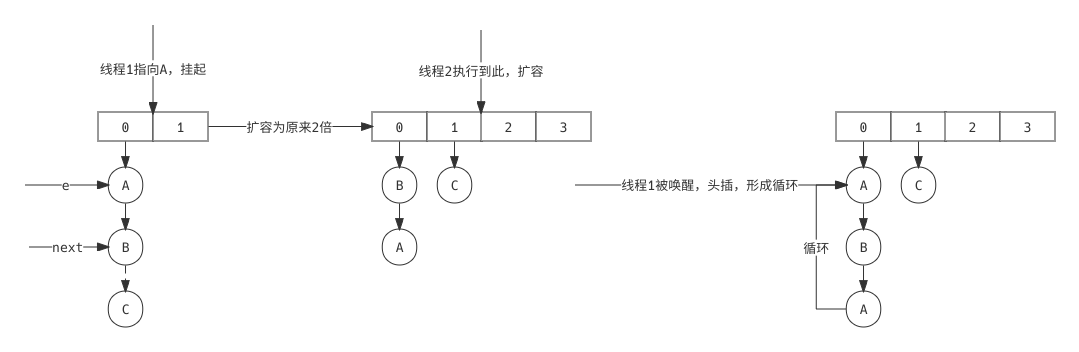### 什么是rehash

rehash主要针对JDK7中的key的hashcode计算规则，在HashMap进行扩容时，元素迁移主要依赖transfer方法实现。

``````void transfer(Entry[] newTable, boolean rehash) {
int newCapacity = newTable.length;
for (Entry<K,V> e : table) {
while(null != e) {
Entry<K,V> next = e.next;
// 这里有一个rehash的判断，在扩容后是否需要对key进行二次hash
// 如果触发了hash函数，由于hashSeed的变化，因此对同一个key的hashcode不一致
// 因此扩容前后key的hashcode也可能变化，根据公式i = hash & (lenght - 1)
// 对于下标的计算同时会受到hashcode和长度的影响
if (rehash) {
e.hash = null == e.key ? 0 : hash(e.key);
}
int i = indexFor(e.hash, newCapacity);
e.next = newTable[i];
newTable[i] = e;
e = next;
}
}
}
``````

transfer传入的参数rehash是initHashSeedAsNeeded方法的返回结果，其入参为扩容后的容量。

``````final boolean initHashSeedAsNeeded(int capacity) {
// 首次扩容时currentAltHashing = false
boolean currentAltHashing = hashSeed != 0;
// VM.isBooted()VM是否启动，结果为true
// Holder.ALTERNATIVE_HASHING_THRESHOLD在类加载时候进行赋值，默认为Integer.MAX_VALUE
// 因此useAltHashing的结果一般为false
boolean useAltHashing = sun.misc.VM.isBooted() &&
(capacity >= Holder.ALTERNATIVE_HASHING_THRESHOLD);
// switching由异或计算得到结果
boolean switching = currentAltHashing ^ useAltHashing;
if (switching) {
// 重新对hashSeed进行赋值，默认为0
hashSeed = useAltHashing
? sun.misc.Hashing.randomHashSeed(this)
: 0;
}
return switching;
}
``````

## 总结

• HashMap是Java集合框架中重要的一员，在JDK7前后有着重大的改变。在JDK8其底层的数据结构实现为数组+链表或者数组+红黑树。频繁的进行扩容会触发元素迁移以及红黑树和链表的操作，会降低HashMap的性能，选择合适的初始化容量可以在一定程度上提升效率。
• HashMap是线程不安全的，如果需要保证线程安全可以使用Collections.synchronizedMap()进行包装，或者使用ConcurrentHashMap。ConcurrentHashMap的粒度是基于分段锁的，其并发性能更好。
• HashMap是基于散列算法的，使用扰动函数可以使其元素分布的更加均匀，查找元素时最理想的时间复杂度是O(1)，在引入红黑树或链表的情况下，查找元素的时间复杂度介于O(1)O(logN)O(N)之间。